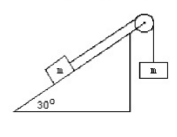# Problem: In the figure below, two masses, each 150 kg, are NOT at rest and are attached to each other by thin unstretchable cable. Find the acceleration of this system of masses and cable. The angle of the ramp is as shown in the figure. (Hint; Draw a free body diagram for each mass) Find the magnitude and direction if the coefficient of friction is 0.100.

###### FREE Expert Solution
81% (297 ratings)
###### Problem Details

In the figure below, two masses, each 150 kg, are NOT at rest and are attached to each other by thin unstretchable cable. Find the acceleration of this system of masses and cable. The angle of the ramp is as shown in the figure. (Hint; Draw a free body diagram for each mass) Find the magnitude and direction if the coefficient of friction is 0.100.Frequently Asked Questions

What scientific concept do you need to know in order to solve this problem?

Our tutors have indicated that to solve this problem you will need to apply the Forces in Connected Objects (Systems) concept. You can view video lessons to learn Forces in Connected Objects (Systems). Or if you need more Forces in Connected Objects (Systems) practice, you can also practice Forces in Connected Objects (Systems) practice problems.

How long does this problem take to solve?

Our expert Physics tutor, Patrick took 9 minutes undefined to solve this problem. You can follow their steps in the video explanation above.